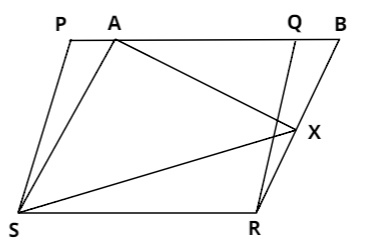Courses
Courses for Kids
Free study material
Offline Centres
More# In the given figure, $PQRS$ and $ABRS$ are parallelogram and $X$ is any point on $BR$. Show that,$(i)$area$(PQRS)$$= area(ABRS),(ii) area(AXS)$$ = $$\frac{1}{2}area(PQRS).AnswerVerified 383.1k+ views Hint: Both the parallelograms are lying on the same base and within the same parallel lines. (i)Consider parallelograms PQRS and ABRS. They are lying on the same base RS and between the same parallel lines RS and PB. And according to the property of parallelogram, we know that parallelograms lying on the same base and between the same parallel lines have equal area. Therefore: \Rightarrow area(PQRS)$$ =$area$(ABRS)$.
$(ii)$Now, consider $\Delta AXS$ and parallelogram $ABRS$. Both are lying on the same base $AS$ and between the same parallel lines $AS$ and $BR$. And we know that the area of the triangle having the base as a parallelogram and its third vertex lying on the opposite parallel side of the parallelogram is half the area of the same parallelogram.
$\therefore$ area$(AXS)$$=$$\frac{1}{2}$ area$(ABRS)$
And we have already proved that, area$(PQRS)$$= area(ABRS). Using this, we’ll get: \Rightarrow area(AXS)$$ =$$\frac{1}{2}$area$(PQRS)$